Mechanics

# Theorem of work and kinetic energy

The work and kinetic energy theorem is very important to the field of mechanics . According to this theorem, the work done on a body is numerically equal to the change in kinetic energy of that body. In addition to being simple to understand, the work and kinetic energy theorem can facilitate the resolution of a large number of exercises.

In order to better understand the meaning behind the work and kinetic energy theorem, it is important to review some important concepts such as kinetic and potential energy.

## What is kinetic energy?

Kinetic energy is a scalar physical quantity, measured in joules according to the International System of Units , which is related to bodies in motion . Every body that has mass and velocity has kinetic energy. The formula used to calculate the kinetic energy is shown below, check it out:C – kinetic energy (J)

m – mass (kg)

v – speed (m/s)

## What is potential energy?

Potential energy is a form of energy related to the position occupied by a body , moreover, all potential energy can be stored in the body. There are several natures of potential energy, however, in the study of mechanics, we focus on the study of gravitational potential energy and elastic potential energy.

• ### gravitational potential energy

Every body that has mass and is at a certain height with respect to the ground is endowed with gravitational potential energy , since it was necessary to consume a certain amount of energy to raise it to that height.

In other words, gravitational potential energy corresponds to the work required to raise a body of mass m to a height h , in a region where the gravitational acceleration is g . Check out the formula used to calculate gravitational potential energy: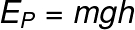m – mass (kg)

g – gravity (m/s²)

h – height (m)

• ### elastic potential energy

Elastic potential energy is the energy associated with the deformation of elastic bodies , such as springs and rubber bands. When an elastic object is stretched or compressed, it tends to return to its original shape. The work done by the elastic force corresponds to the elastic potential energy. This energy depends on the deformation of the body, but also on its spring constant k . Note the formula that is used to calculate elastic potential energy: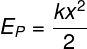k – spring constant (N/m)

x – deformation of the body (m)

## work of a force

The work of a force is the term that designates the transfer of energy to a body through the application of a force . The work of a constant force is calculated by the product of the force and the distance traveled by the body: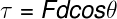τ – work (J)

F – force (N)

d – distance (m)

cosθ – cosine of the angle between the force f and the distance d

If the force exerted on the body is not constant, the work exerted on it can be obtained from the graph that relates the force to the distance covered. In this case, the work corresponds to the chart area .

## Formula of work and kinetic energy

The formula used for the work and kinetic energy theorem is represented in the following figure, note: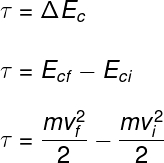ΔE C – change in kinetic energy

cf e ci – final and initial kinetic energy

f e i – final and initial speed (m/s)

## Exercises solved

Question 1 – Consider an object that started from rest and has its speed increasing, moving without friction and under the action of a single force. Suppose its kinetic energy, after time t since its departure, is E and, at time 2t, is 4E. Regarding the work done by the force acting on the object, it is correct to state that:

A) is worth 3E during the interval between t and 2t.

B) is zero, since there is only change in kinetic energy.

C) is worth 5E during the interval between t and 2t.

D) it is not possible to be determined, as there is no information about the value of the force or about the displacement.

Template: Letter A

Resolution

According to the work and kinetic energy theorem, the work done on a body is equal to its change in kinetic energy, therefore, between the time intervals t and 2t, as the kinetic energy of the body changed from E to 4E, the work exerted on it will be 3E, so the correct alternative is the letter A .

Question 2 – The first Brazilian geostationary satellite was launched into space in 2017 and will be used for strategic government communications and to expand the offer of broadband communication. The rocket that took the satellite into space was launched from the Kourou Space Center in French Guiana. The satellite’s mass is constant from launch to orbit entry and is m = 6.0 x 10 3 kg. The magnitude of its orbital speed is v or = 3.0 x 10 3 m/s.

Neglecting the initial speed of the satellite, due to the rotation of the Earth, the work of the resultant force on the satellite to bring it to its orbit is equal to:

A) 2 MJ

B) 18 MJ

C) 27 GJ

D) 54 GJ

Template: Letter D

Resolution

The work done on the satellite is equal to its change in kinetic energy, so: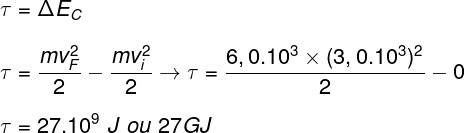Based on the calculation made, the correct alternative is the letter D